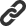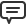# Blog

All Blog Posts  |  Next Post  |  Previous Post

#Symbolic integration in TMS Analytics & Physics 3.0

Bookmarks:

#### Tuesday, September 10, 2019

The main new feature of TMS Analytics & Physics 3.0 is symbolic integration. The version allows calculate symbolic expressions of indefinite and definite integrals and analytically evaluate definite integrals on the specified intervals.
Symbolic integration can be implemented with very simple code. Here is the example of typical code template to get symbolic expression of an indefinite integral:

```var
f, i: string;
begin
f:= ...;

try
i:= translator.Integral(f, 'x');
except
on ex:Exception do ; // handle the exception ‘ex’
end;

// using symbolic expression ‘i’ of the integral
end;
```
Here ‘translator’ is an instance of the TTranslator class, which realizes all methods for symbolic integration; ‘f’ is the function to integrate. The ‘Integral’ method calculates analytical expression of indefinite integral by ‘x’. Assigning various expressions to the ‘f’ function, we get symbolic expressions of the integrals:
```f = ‘(A*x+1)^(1/3)+1/(2-x/B)^(2/3)’
i = ‘3/4*(A*x+1)^(4/3)/A-(2-x/B)^(1/3)*3*B’

f = ‘(e^x-e^-x)*x^2-A*B^(x/2-1)’
i = ‘x^2*e^x-2*(x*e^x-e^x)+x^2*e^-x-2*(-x*e^-x-e^-x)-A*B^(x/2-1)/ln(B)*2’

f = ‘2*sin(x)-B*cos(a-x/2)+tan(x/2)^2’
i = ‘-2*cos(x)+B*sin(a-x/2)*2+2*(tan(1/2*x)-1/2*x)’
```
It should be noted here that symbolic integration can handle not all math expressions. For example, not all math operators allow analytical integration. If the method fails to calculate some integral – special exception with according message will be thrown. There is another overloaded version of the ‘Integral’ method that allows calculating symbolic expressions for definite integrals. The code for the case is the following:
```var
f, x1, x2, i: string;
begin
f:= 'A*e^x-sin(x)/2';
x1:= 'Pi';
x2:= 'sin(y)';

try
i:= translator.Integral(f, 'x', x1, x2);
except
on ex:Exception do ; // handle the exception ‘ex’
end;

// using symbolic expression ‘i’ of the definite integral
end;```
Here ‘x1’ and ‘x2’ are the lower and the higher limits of definite integral. The method ‘Integral’ uses Newton-Leibniz axiom for calculating definite integrals. For the specified integrand f=’A*e^x-sin(x)/2’, symbolic expression of the definite integral is i=’A*e^sin(y)+1/2*cos(sin(y))-A*e^p-1/2*cos(p)’.

Note that the integration limits must not depend on the integration variable (‘x’ in the examples). The limits can contain other variable names, functions, operators and other expressions. If the limits are constant and no other variable used in the integrand, then the result expression can be simplified. For example, using f=’2*x^2’, x1=’3’, x2=’5’ in the code above, we get i=’196/3’. Nevertheless, the result value is symbolic expression. To evaluate definite integrals (calculate numerical value), one must use the ‘Integrate’ method of the TTransaltor class as in the following code:
```var
f, x1, x2: string;
iv: TValue;
i: TFloat;
begin
f:= '2*x^2';
x1:= '3';
x2:= '5';

try
iv:= translator.Integrate(f, 'x', x1, x2);
i:= iv.AsType();
except
on ex:Exception do ; // handle the exception ‘ex’
end;

// using float value ‘i’ of the definite integral
end;
```
Now i=65.3333333333333 and it is float value of the definite integral.

Note that when evaluating definite integrals the integrand expression can contain other variable or even functions of other variables. However, these variables must be added to the translator instance for the evaluation process. For example, adding the following variables
```  translator.Add('A',-1);
we can evaluate definite integral for f=’sin(n*x)+e^(x/n)’, x1=’A’, x2=’B’ and getting the value i=3.04355393035906.

TMS Analytics & Physics library realizes base integration rules (sum rule, multiplication by constant and so on); provides default integrators for all algebraic, base special and transcendental functions; realizes integration by-parts algorithms for special expression types. The library also allows implementing user-defined integrators for special cases of functions and expressions. Thus, the functionality of symbolic integration can be easily extended.

The version 3.0 is already available. Source code of the demo project for the article can be downloaded from here.

Bruno Fierens

Bookmarks: